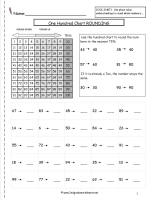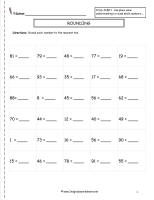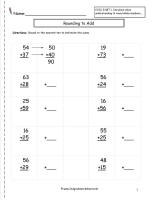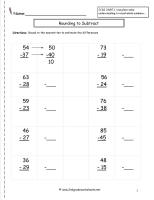# Rounding Whole Numbers, Rounding to Add and Subtract

## Rounding Whole Numbers Worksheet, Rounding to Add and Subtract.

###One Hundred Rounding Chart Worksheet

Common Core State Standards: CCSS 3.NBT.1 Use place value understanding to round whole numbers.....
Guide chart for rounding to the nearest ten.

###One Hundred Rounding Chart Worksheet2,3

Common Core State Standards: CCSS 3.NBT.1 Use place value understanding to round whole numbers.....
Students round whole numbers to the nearest ten. Guide chart for rounding to the nearest ten included.

###Rounding to the Nearest Ten Worksheet2,3

Common Core State Standards: CCSS 3.NBT.1 Use place value understanding to round whole numbers.....
Students round whole numbers to the nearest ten.

###Rounding to Estimate the Sums2,    3

Common Core State Standards: CCSS 3.NBT.1 Use place value understanding to round whole numbers.....
Students round whole numbers to the nearest ten to estimate sums.

###Rounding to Estimate the Differences

Common Core State Standards: CCSS 3.NBT.1 Use place value understanding to round whole numbers.....
Students round whole numbers to the nearest ten to estimate differences.